# Changing Summation Limits

Previous: Sigma Notation Properties

Next: Example: Changing the Summation Limits

In some cases we need to find an equivalent representation of a given summation, but that has different summation limits. For example, we may need to find an equivalent representation of the following sum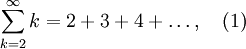where the index of summation start at 1 instead of 2. We will introduce two methods for doing this.

## Method 1

Introducing the transformation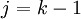, so that when k = 2, j = 1, yields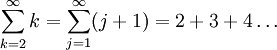as desired. The summation index now starts at 1 instead of at 2.

### Method 1 Observations

If we like, we can go back to calling our summation index k,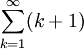because it does not matter what we call our index. Also observe that the transformationwas chosen so that our new index of summation,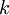, starts at 1. You will also notice that although we accomplished our task, the general term was transformed from k to k + 1. This may not be what we need in certain problems, as we will see in other lessons within this Unit. Method 2 requires more work, but circumvents this problem.

## Method 2

Now suppose we would like to

• re-write the sum so that we have the index of summation start at 1, but
• not change the general term.

Instead of using a change of variable, we can use another trick to accomplish this task.

Our procedure is to add and subtract terms in the sum to shift our index to 1: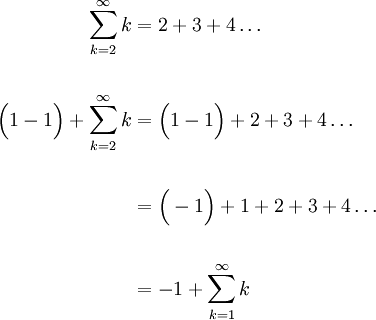Therefore,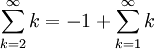as desired. Using Method 2, the general term (in this example the general term is k) has not changed.

If you find this process confusing or wonder why we would need to use it, fear not. We will go through another example in more detail, and additional lessons in this unit make use of this method.

Previous: Sigma Notation Properties

Next: Example: Changing the Summation Limits